# Discrete time survival models in python

Sorry in the advance for the long post! I’ve wanted to tackle a project on estimating discrete time survival models for awhile now, and may have a relevant project at work where I can use this. So have been crunching out some of this code I am going to share for the last two weeks.

I personally only have one example in my career of estimating discrete time models, I used discrete time models to estimate propensity scores in my demolitions and crime reduction paper (Wheeler et al., 2018), since the demolitions did not occur at all once, but happened over several years. (In that paper I estimated the discrete time models, and then did matches in random cohorts.)

But I was interested in discrete time survival models for one reason – they allow you to estimate very non-linear hazard functions that you cannot with traditional survival models. For Cox models, to do predictions you need to rely on a estimate of the baseline hazard function, and for parametric models (e.g. Weibull) they often can only have monotonic or flat functions (so can’t be low risk and then high risk in a short period). For a good reference about evaluating predictions for survival models, I suggest Haider et al. (2020), and for a general reference for discrete time survival models I suggest the little Sage green book by Paul Allison (Allison, 2014).

For traditional recidivism studies in criminology (e.g. after someone is paroled), I don’t believe the function to be too bumpy like this, so I don’t think prior studies are misleading (e.g. Denver, 2019). But I do think they are worth examining to see if that is the case. For another use case, for chronic offender based police predictions, I think individuals may have more bumpy risk profiles, e.g. you commit a crime and then lay low (so lower risk), or get victimized and may want retaliation (so high risk). A prior work I looked at a year horizon for offender predictions (Wheeler et al., 2019), so I wanted to extend that to shorter time intervals, but never quite got the chance. (Another benefit of discrete time models is that they can incorporate time varying factors no problem the way the model is set up.)

I have code illustrating discrete time models saved on github here. The data I use to illustrate the analysis is taken from Ruderman et al. (2015). This is recidivism for a fairly large cohort. (I don’t think discrete time makes much sense for small samples, you probably need 1000+ to even really consider it I would guess.)

The code ends up being too long to walk though in a blog post. So here are some quick notes/tables/plots, and I encourage you to go check out the github page to dive deeper if you want.

# The Discrete Time Model Setup

The main thing to realize about the discrete time modeling set up is that you just turn your survival data problem into a format you can leverage logistic regression (or whatever binary prediction machine learning model you want). So if we have an original set of survival data that looks like:

``````ID Time Outcome
A   4    1
B   3    0``````

We then explode this dataset into a long format that looks like this:

``````ID Time Outcome
A   1     0
A   2     0
A   3     0
A   4     1
B   1     0
B   2     0
B   3     0``````

So you can see ID A was exploded to 4 observations, and the Outcome variable is only set to 1 at the final time period. For person B, they are exploded 3 observations, but the outcome variable is always set to 0.

Then you model Outcome as a function of time and other covariates, which can be either constant per person or time varying. This then gets you a model that estimates the instant probability of death (or failure) in a particular time sliver. The way I think about it is like this – we can predict whether you will commit a crime sometime within the next week (the cumulative probability over the entire week), or within a particular sliver of time (the probability of committing a crime Friday at 10 pm). Discrete time models pick a sliver of time, e.g. Friday, and calculate the instant probability within that bin.

But then we don’t want to rely on the traditional binary metrics to evaluate this model – we will often want to go from the instant probabilities in a time sliver to the cumulative probabilities. You can take those model estimates though at aggregate them back up to examine over the weekly time horizon example though. So if we have predictions for a new person C that looked like this:

``````ID Time InstantProb
A   1     0.2
A   2     0.1
A   3     0.3
A   4     0.05``````

We could then calculate the cumulative probability of failure over these four time periods. So the failure in time period 1 is just `0.2`. For time period 2 it is `1 - [(1-0.2)*(1-0.1)] = 0.28`. You just then accumulate those individual specific probabilities into cumulative failure probabilities over particular time horizons, which you can then incorporate into cost-benefit analysis for how you will use those predictions in practice. For various metrics we will then examine not just the instant probability our model spits out, but also the cumulative probability of failure.

The main issue with these models is that when exploding the dataset it can result in large samples. So my initial sample of just over 13k observations, when I expand to observed weeks ends up being over 1 million observations. That is not a big deal though, I can still easily do whatever models I want with that data on my personal machine. Probably don’t need to worry about it for most statistical computing projects until maybe you are dealing with over 20 million observations I would bet for most out of the box desktop computers anymore.

# Modeling Notes

In the github page the script `00_PrepData.py` prepares the dataset (transforming to the long format). The original Ruderman data has repeated events, but for simplicity I only take out the first events for individuals, which ends up being just over 13k observations. I then split this into a training dataset and a test dataset, and a set the test dataset to 3k cases.

My temporal unit of analysis I transform into weeks since release, and only examine the discrete time models up to 104 weeks (so two years). Here is a traditional KM plot based on the exploded discrete time training dataset.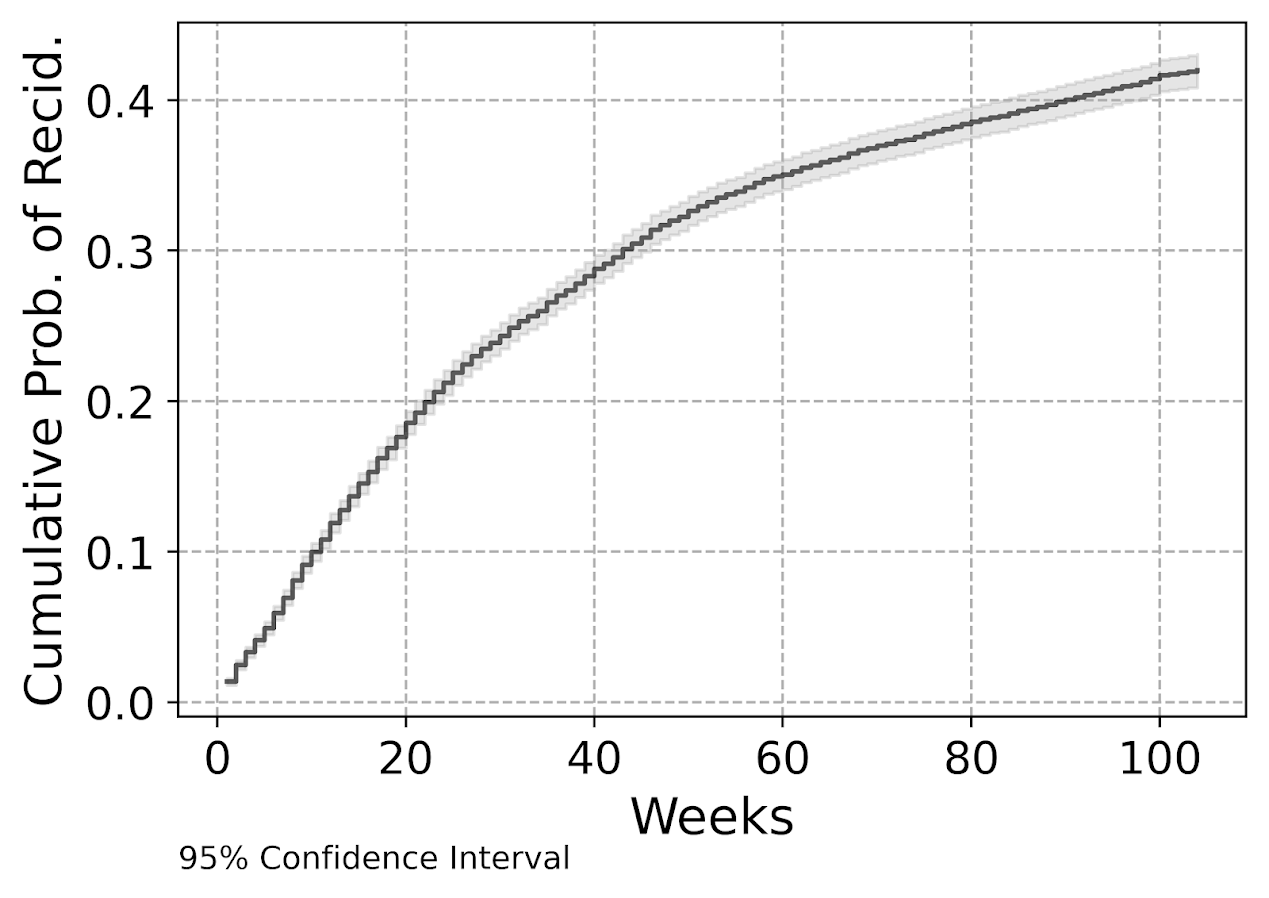But really what we are modeling in this set up is the instant hazard, not the cumulative hazard. So here is a plot of the instant probability of recidivism.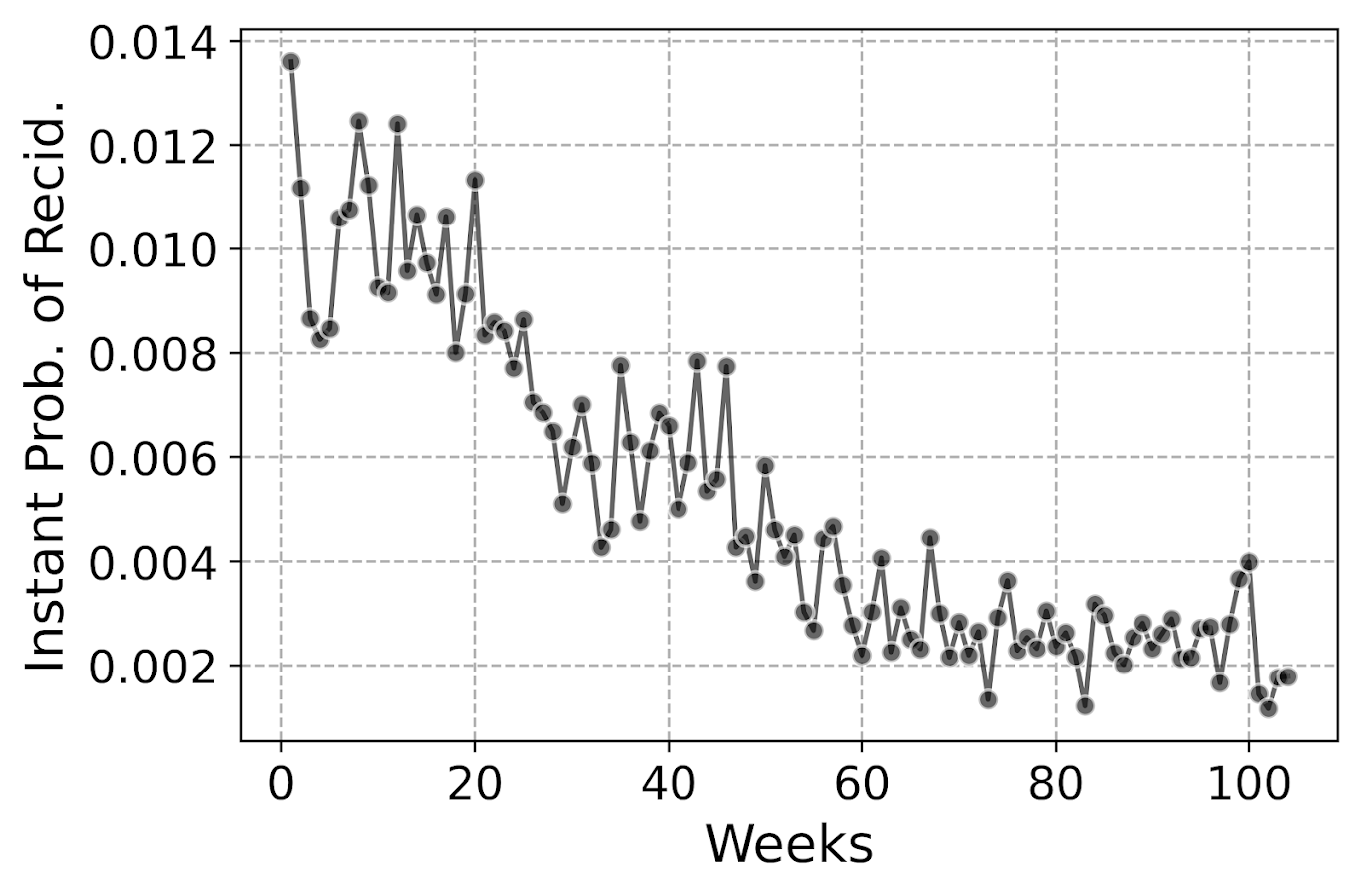You can see that in the first week out, almost 1.4% of the individuals recidivate. There are ups and downs, but the instant probability continues to decrease and slightly flatten out out to 100 weeks. So you can see how over those two years we go from an original dataset of over 10k to around 3k due to censoring.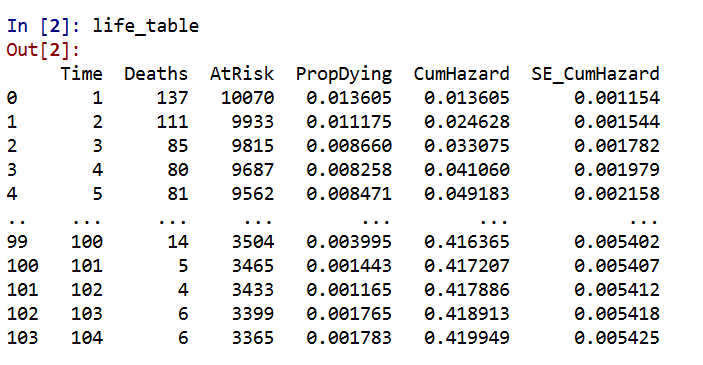Part of the reason I was interested in examining discrete time models is that I was wondering if the instant hazard was bumpy and had some ups and downs when people are first exposed.

But this data appears fairly smooth, so in the end I end up fitting a logistic regression model with restricted cubic splines for time with knot locations at `[4,10,20,40,60,80]`. I also incorporate various interactions with the some of the time invariant covariates in the original Ruderman data (age at first arrest, male, overcrowding, concentrated disadvantage index, and offense category dummies).

I initially tried my GoTo machine learning models of random forests and XGBoost, but they performed quite poorly. Tree based models aren’t very well suited to estimate very tiny probabilities I am afraid. So that will need some more tinkering to see if I can use those machine learning models more effectively in this circumstance. I’m wondering if doing a different loss function makes sense (so do the loss based on the cumulative hazard instead of the instant). Here also I did not regularize the logit model, but with time varying factors that may make sense.

The Haider paper looks at the R MLTR package, which is similar to here but slightly different, in that they are modeling the cumulative hazard directly instead of the instant hazard. (So instead of chopping off the 1’s and the end of the vector, you keep padding them on for observations.) So in that case you want to enforce monotonic constraints on the time effect.

# Checking Out Individual Predictions

The remaining sections in the blog post are all taken from the second `01_EvalTime.py` script. So first, after you generate your predictions on the training data, you can then pull out a particular individual and check out our predictions for their cumulative survival probability based on our predictive model. The red line shows that this individual actually recidivated at 45 weeks, in which their cumulative risk was just above 20%.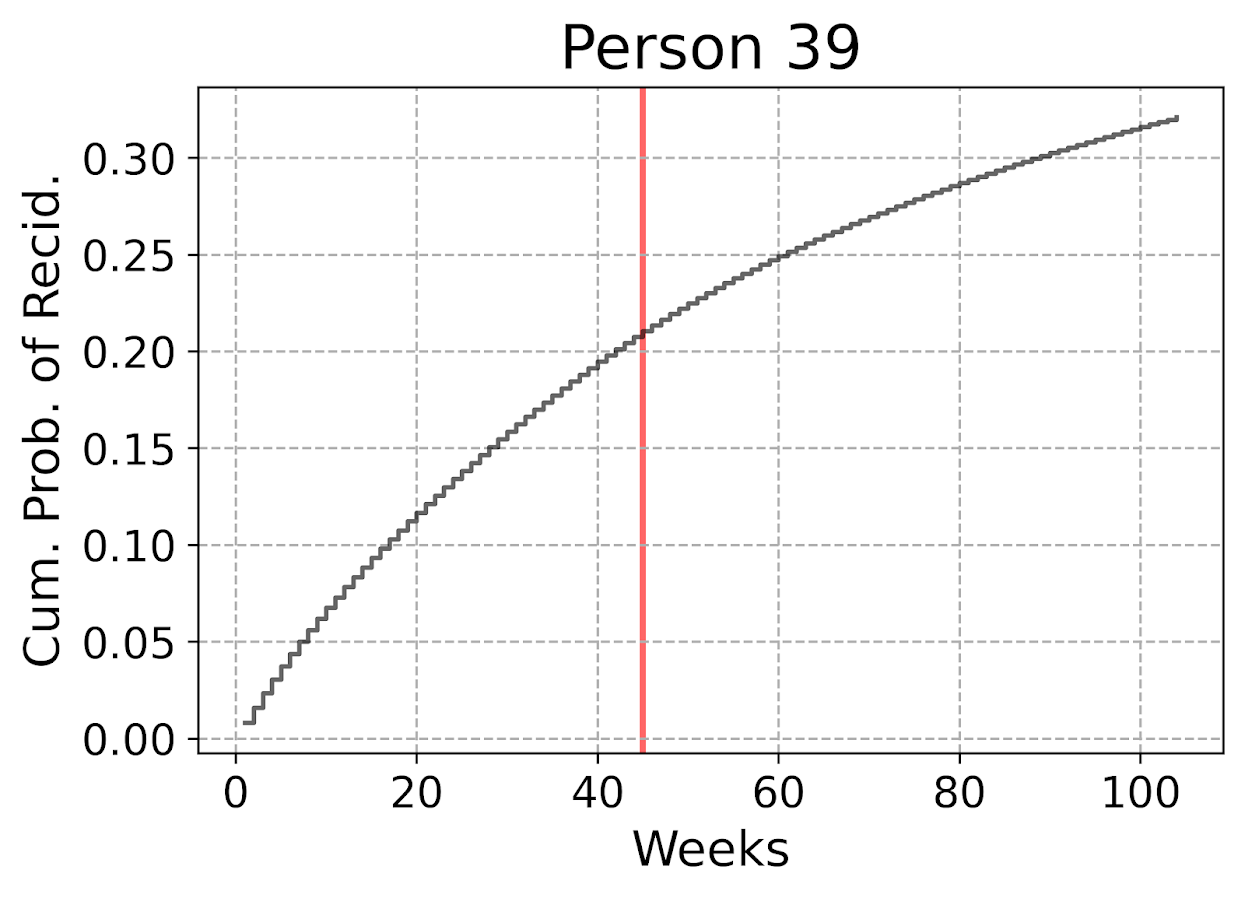The cumulative probability will never be super interesting though – in that even if you had a very wiggly instant hazard the cumulative hazard is always monotonically increasing. So if you check out the instant hazard this will show how a persons risk level varies over time.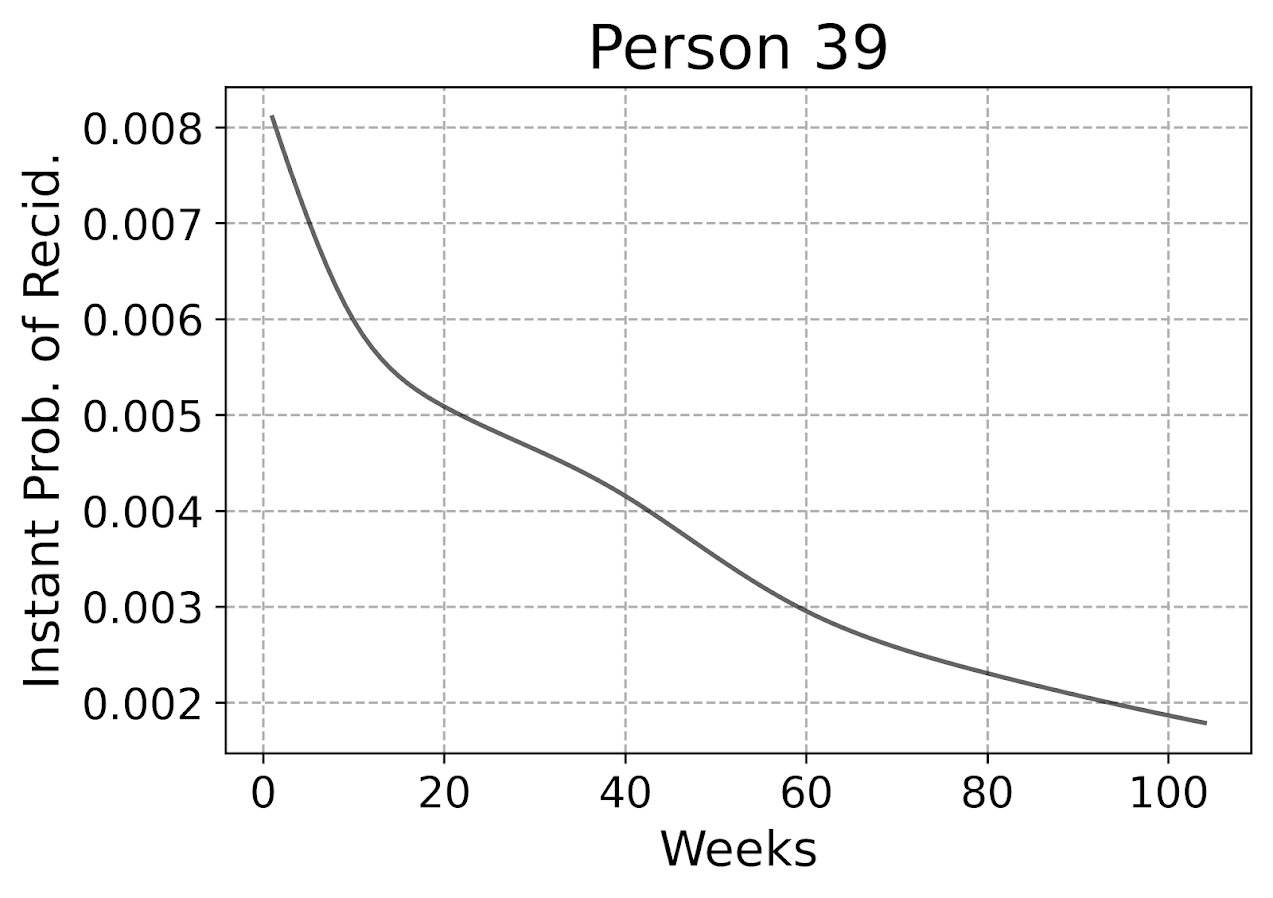So we can see here that person 39 had a predicted high risk when they are first released, but gradual decreases in a few steps over time. The way I have modeled this using restricted cubic splines it has to be smooth, but you could say incorporate dummy variables for the first 10 weeks, in which case this prediction could be quite bumpy.

Given this always shows monotonically decreasing hazard, you wouldn’t be able to exactly fit that function using parametric models, but they would be not too far off. So this dataset doesn’t appear to be a real great showcase of the utility of discrete time models!

But doing some plots of the instant hazards may be interesting to try to identify particular different risk profiles, or maybe even use some clustering (like group based trajectory models) to identify particular latent risk profiles. (It may be most people are smoothly decreasing, but some people have bumpier profiles.)

# Evaluating Model Calibration

Haider et al. (2020) break down predictive metrics to evaluate survival models into two types: calibration is that the model predictions match actual cases, e.g. if my model says the probability of failure is 20%, does the data actually show failure in 20% of the cases. The other is discrimination, can I rank individuals as high risk to low risk, and do the high risk ones have the negative outcome more frequently.

While the Haider paper has various metrics, I am kind of confused how to do them in practice. My confusion mostly stems from the test dataset will ultimately have censoring in it as well, so the calibration metrics need to take this into account. Here are my attempts at a few plots that take on the task of checking model calibration.

First, I’ve previously discussed what I call a lift calibration chart. I adjust it here though to account for the fact that we have interval censoring, and I create ignorance bounds for the actual proportion of failures in the dataset.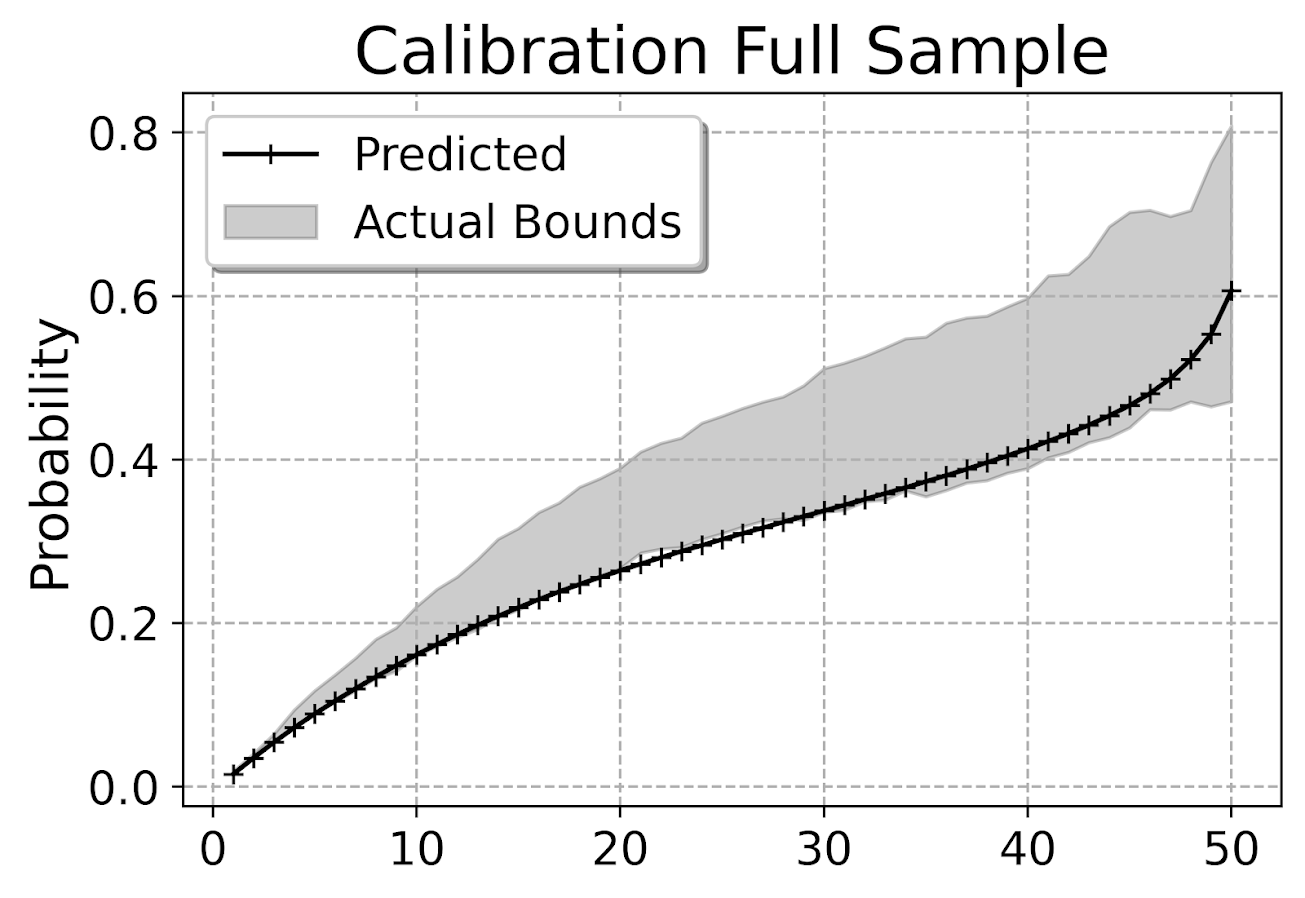This is for the full sample, which I expanded out and did calculations for up to 104 weeks for everyone. You can do a slice of the data though for a particular time period and check the same calibration. So here is an example checking calibration at one year out.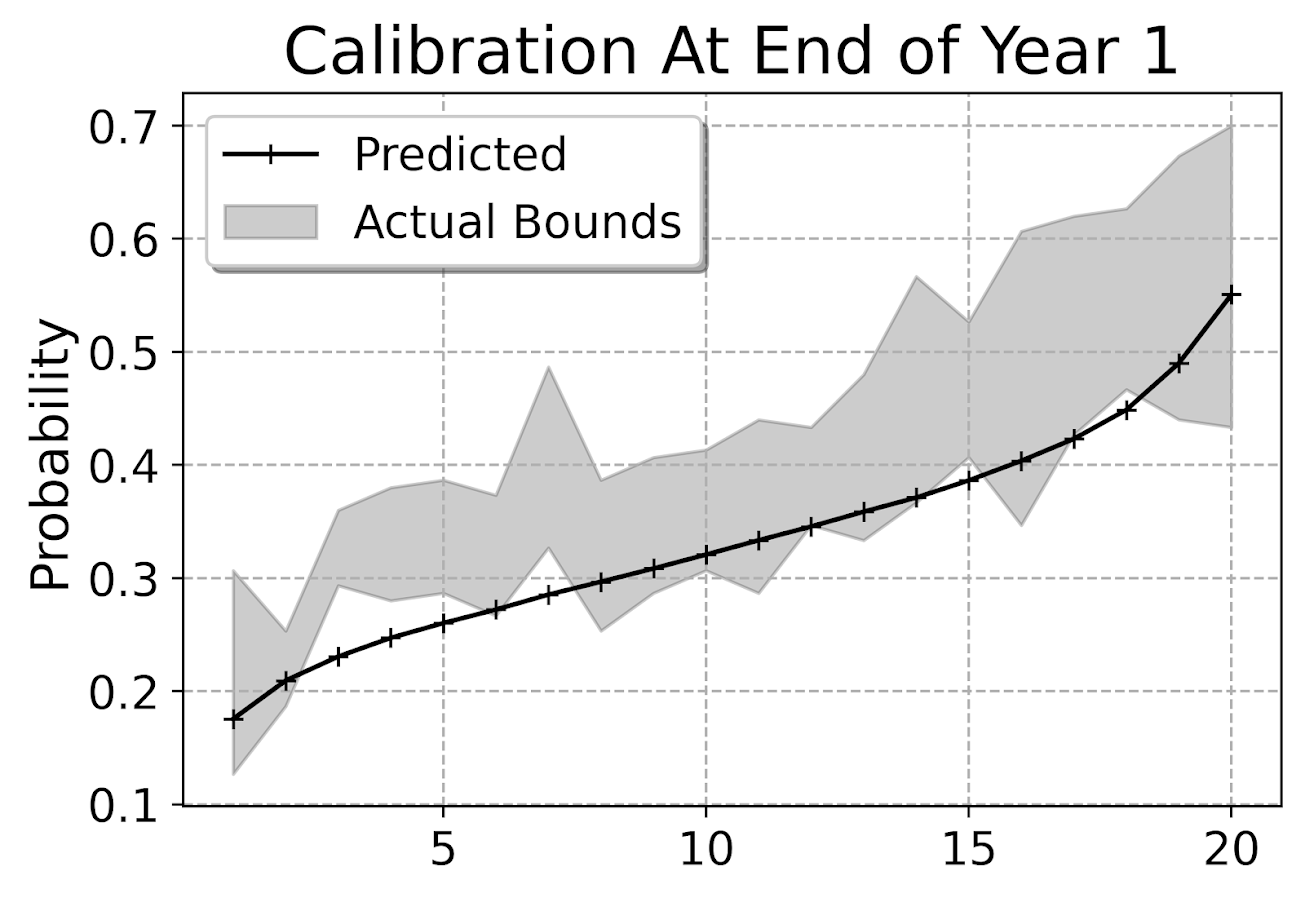The earlier in time the smaller the ignorance bands will be (as there will be less censoring in sample). Here is what the created dataset looks like to illustrate how the ignorance bands are calculated.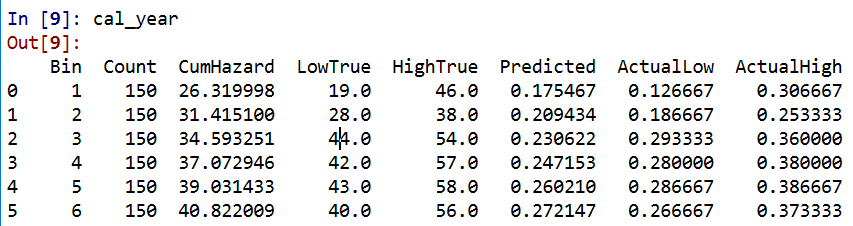The `CumHazard` column is my predicted line, which I break down into 20 bins for that yearly plot (so with 3000 training dataset observations, results in bins of 150 observations). Then you can see the `LowTrue` column (in Bin 1) signifies I observed 19 failures in that set of observations, but there ending up being a total of 27 observations censored in that bin, `46 - 19`. So the actual proportion in the data could either be 19/150 (all censored never recidivate) or 46/150 (all of those who were censored would end up recidivating). I would suggest for notes on ignorance bounds like these (which also apply to ECDF type functions), Ferson et al. (2007).

I’d note that this is the same way you generate data for a Hosmer-Lemeshow test for logit models, but I don’t bother with the Chi-Square test. For large samples it will always reject, and small samples it may mean you just have low power, not that your model is well calibrated. So doing that stat test is a lose-lose IMO. But you can just make the plot to see whether your predictions are on the mark, or if they are low or high on average. Here we can see that they hug the lower ignorance band, so are not too bad. But may be a shade too low (more people recidivate than predicted).

This calibration is examining the probability, but another way to think about calibration here is calibrated in terms of time, e.g. I say something will happen in 30 weeks, does it actually happen in 30 weeks? Here is my attempt at a plot to check that out. Using the test dataset, I generate the usual KM estimate. Then based on the predicted probabilites, I generate simulated outcomes for individuals (here 99), and then plot the range of those outcomes on the same chart.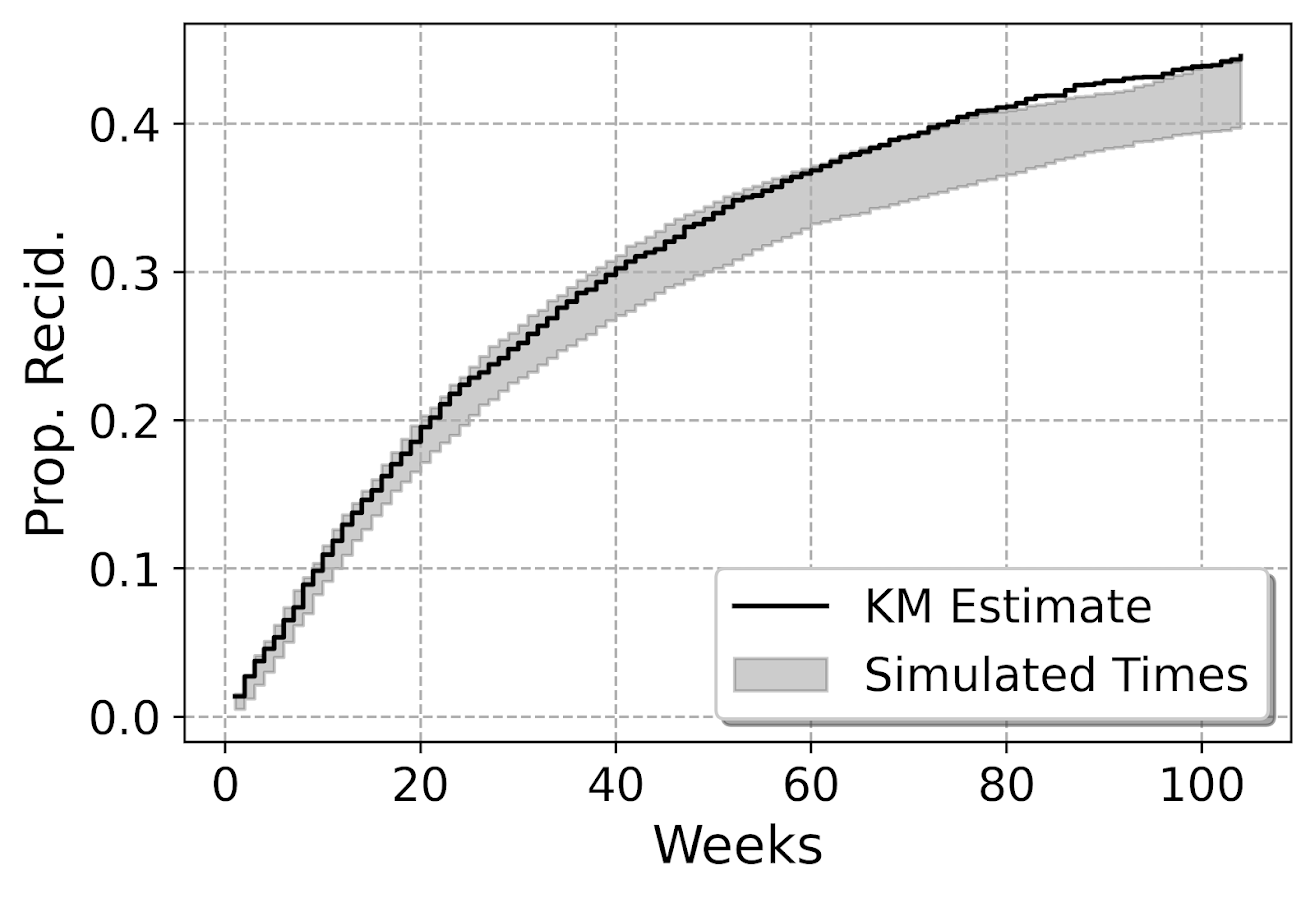So here you can see that my predicted failure times are somewhat longer than observed in the data (simulation bands slightly below observed for the later time periods). These two charts are likely not in contradiction, the error bands in each show somewhat observe patterns, so they both hint at my model is conservative in assigning risk. But it is not too shabby in terms of calibration (you should have seen some of these plots when I was trying random forest and XGBoost models!).

I’m wondering offhand if I have some edge effects going on. So maybe even if I am only interested in examining a time horizon of two years, I should still tack on longer time periods for the initial models.

Both of these charts you can subset the data and look at the same chart, so here is an example table generated for simulations based on 332 test dataset females. Because the sample is smaller, the simulated bands are wider, so the observed KM cumulative hazard estimate appears well inside the bands here for the female subsample. (Probably because of less diagnostic ability to identify tiny bits off in the calibration.)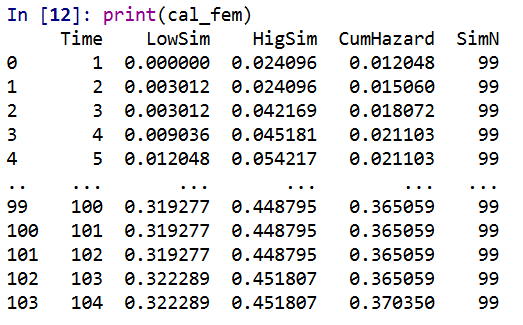# Evaluating Model Discrimination

The second way you might evaluate survival predictions is in terms of rankings, can I discriminate in my model between individuals who are high risk and who are low risk. One of the crazy things about these individual level survival curves is that they can cross! So imagine we had a set of two individuals and are looking at a horizon of four periods:

``````ID Time InstProb CumProb
A   1      0.1     0.1
A   2      0.1     0.19
A   3      0.1     0.271
A   4      0.1     0.3439

B   1      0.2     0.2
B   2      0.1     0.28
B   3      0.05    0.316
B   4      0.01    0.32284``````

So person B is at higher risk right away. So if we ranked these individuals for who was more likely to recidivate, ID B will be ranked higher for periods 1, 2 and 3. But by period 4, ID A is at higher risk in terms of their cumulative probability of recidivating.

The simplest metric to evaluate discrimination IMO is AUC (which is related to the concordance metric). And to do that you just do slices of particular weeks, and then calculate the AUC based on the cumulative failure probability estimate at that time period.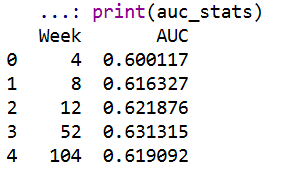So you can see here that it is pretty meh – only AUC stats around 0.6 for my logit model. So better than the random 0.5, but not by much. Even though my model appears to be reasonably calibrated, it is nothing to brag to grandma about being able to identify people at different risk levels for recidivism, not matter the time horizon I am interested in.

For this estimate I just dropped censored observations, so I am not sure how to deal with them in this case. If you have suggestions or references let me know! But offhand I don’t think they are too off, the earlier time periods should have less censoring, but they are all pretty close in terms of the overall metric.

# Future Stuff?

Besides seeing how others have dealt with censoring in their prediction metrics, another metric introduced in the Haider et al. (2020) paper is a Brier Score that is both a calibration and discrimination metric.

Also for folks interested in survival analysis in python, I suggest to check out statsmodel or the lifelines packages.